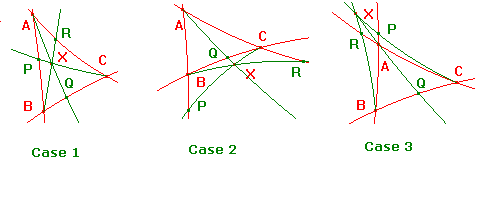# Proof of Ceva's Theorem

 Ceva's Theorem for Hyperbolic Triangles If X is a point not on any side of an h-triangle ABC such that AX and BC meet in Q, BX and AC in R, and CX and AB in P, then h(A,P,B)h(B,Q,C)h(C,R,A) = 1. Proof A little consideration shows that, if we change the labels of the vertices, then either the factors are simply permuted, or are inverted and permuted. Since our aim is to show that the product is 1, the labelling of the vertices of the h-triangle is immaterial. It follows that there are essentially three cases, depending upon the position of X relative to A. These are illustrated below.Case 1 Applying Menelaus's Theorem to the h-triangle AQC, cut by BXR, |h(A,X,Q)h(Q,B,C)h(C,R,A)| = 1,so that (sinh(d(A,X)/sinh(d(X,Q))(sinh(d(Q,B)/sinh(d(B,C))(sinh(d(C,R)/sinh(d(R,A)) = 1. Similarly, applying Menelaus's Theorem to the h-triangle AQB, cut by CXP, (sinh(d(A,X)/sinh(d(X,Q))(sinh(d(Q,C)/sinh(d(C,B))(sinh(d(B,P)/sinh(d(P,A)) = 1. Dividing these two expressions and cancelling common factors, we get (sinh(d(A,P)/sinh(d(P,B))(sinh(d(B,Q)/sinh(d(Q,C))(sinh(d(C,R)/sinh(d(R,A)) = 1. Since, in this case, the sides are cut internally, h(A,P,B)h(B,Q,C)h(C,R,A) = 1, as required. Cases 2 and 3 are almost identical, with just the signs of the h(.,.,.) to be taken into account. In each case, two of the cuts are external, so the product of the h(.,.,.) is still positive. Note The proof is essentialy the same as for the euclidean case, with the appropriate definition of signed ratio being used. return to ceva and menelaus page